# Upper Triangular Matrix Column Major Mapping

## Upper Triangular Matrix by Column-Major Mapping in C and C++:

In this article, I am going to discuss Upper Triangular Matrix by Column-Major Mapping in C and C++ Language with Examples. Please read our previous article, where we discussed Upper Triangular Matrix by Row-Major Mapping in C and C++ Language with Examples.

##### Upper Triangular Matrix by Column-Major Mapping:

Now let us look at column-major mapping. We have to store an upper triangular matrix in a single dimension array column by column.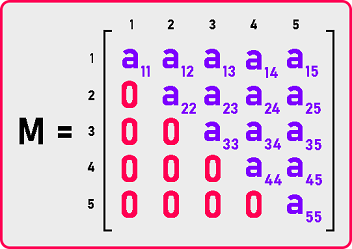This is our upper triangular matrix. So non-zero elements are in the upper triangular part of this square matrix. a12, a13…, a55 are non-zero elements. ‘i’ is the raw numbers and ‘j’ is the column numbers. But which elements are non-zero? We have a condition for finding non-zero and zero elements which are: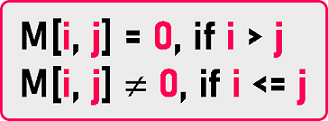So, if i is less than or equal to j then the elements will be non-zero. If i is greater than J then the elements will be zero.

Total number of non-zero elements is:

= 5 + 4 + 3 + 2 + 1, means,

= n + (n-1) + (n-2) + … + 3 + 2 + 1

= n * (n + 1) / 2.

Total number of zeros elements is:

= total no. of elements – no. of non-zero elements

= n2 – n (n + 1) / 2

= n (n-1) / 2

This is how we can define our upper triangle matrix. Now how do we present this one in a single dimension array? We will not be taking a two-dimensional array and filling it with these many zeros. We have to avoid storing zeros so that we can save space and processing time.

So, what should be the size of the array? The size will depend on the number of non-zero elements. As we calculate no. of non-zero elements, so the size will be n * (n + 1) / 2. Let us create a single dimension array: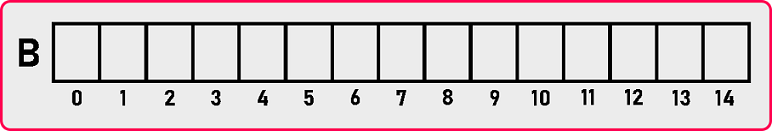Now let’s see Column Major mapping: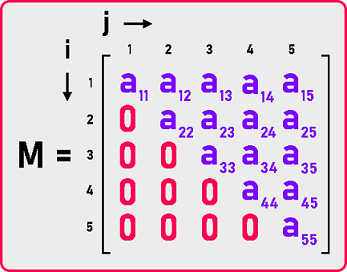Now we will map the matrix column by column in the single dimension array as:

###### 1st column: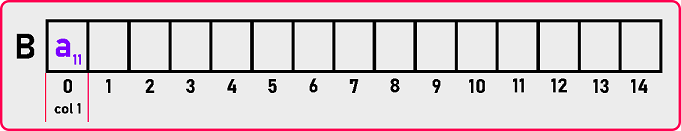###### 2nd column: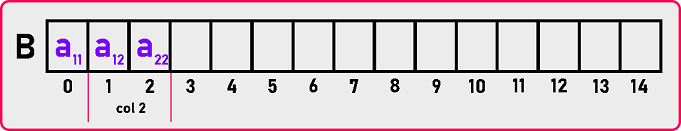###### 3rd column: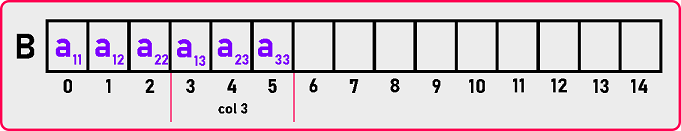###### 4th column: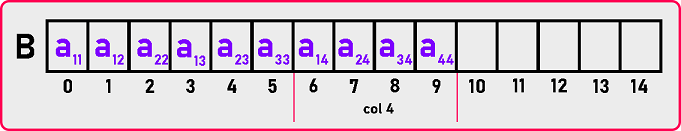###### 5th column: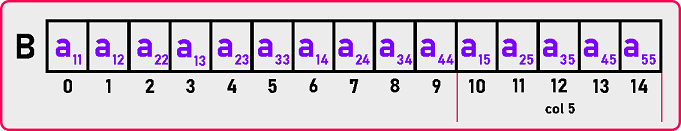In this way, all these elements will be mapped to this array. Now we need a formula so for formula let us take one example and observe it.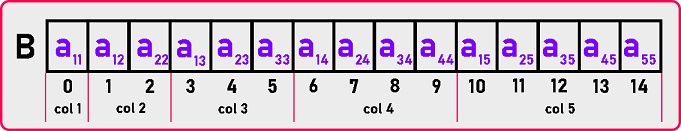Let us take one example and see. We want to find the indices of M . M  means the element which is present in the 4th row and 5th column. As we can see in the array, M  is present on the 13th index. The element is present in the 5th column. So, we need to skip the 1st, 2nd, 3rd, and 4th columns to reach the 5th column.

1. 1st column having 1 element: Index (M ) = 1 +
2. 2nd column having 2 elements: Index (M ) = 1 + 2
3. 3rd column having 3 elements: Index (M ) = 1 + 2 + 3
4. 4th column having 4 elements: Index (M ) = 1 + 2 + 3 + 4

Now after skipping the 4 columns, we are on the beginning of 5th column: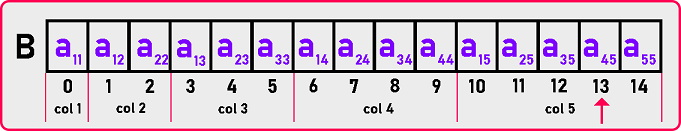Now how much we should move ahead to reach a45? 3 elements. So,

Index (M ) = [1 + 2 + 3 + 4] + 3

Index (M ) = 13

Here we got the 13th index. Let’s create the formula by observing the above example:

Index (M ) = [1 + 2 + 3 + … + (j – 1)] + (i – 1)

Index (M ) = [ j * (j – 1) / 2] + (i – 1)

This is the required formula for Column-Major Mapping of Upper Triangular Matrix. Now let see the code part:

##### Upper Triangular Matrix by Column-Major Mapping Code in C Language:
```#include <stdio.h>
#include <stdlib.h>
struct Matrix
{
int *B;
int n;
};

void Set(struct Matrix *m, int i, int j, int y)
{
if (i <= j)
m->B[j * (j - 1) / 2 + (i - 1)] = y;
}

int Get(struct Matrix m, int i, int j)
{
if (i <= j)
return m.B[j * (j - 1) / 2 + (i - 1)];
else
return 0;
}

void Display(struct Matrix m)
{
int i, j;

printf ("\nMatrix is: \n");

for (i = 1; i <= m.n; i++)
{
for (j = 1; j <= m.n; j++)
{
if (i <= j)
printf ("%d ", m.B[j * (j - 1) / 2 + (i - 1)]);
else
printf ("0 ");
}
printf ("\n");
}
}

int main()
{
struct Matrix M;
int i, j, y;

printf ("Enter Dimension of Matrix: ");
scanf ("%d", &M.n);
M.B = (int *) malloc (M.n * (M.n + 1) / 2 * sizeof (int));

printf ("Enter all the elements of the matrix:\n");
for (i = 1; i <= M.n; i++)
{
for (j = 1; j <= M.n; j++)
{
scanf("%d", &y);
Set(&M, i, j, y);
}
}

Display (M);
}
```
###### Output: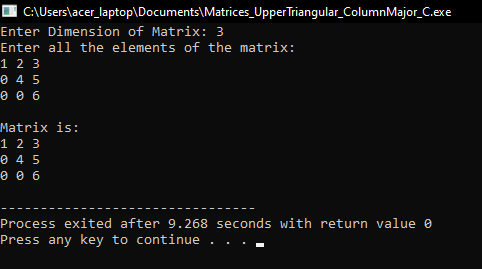##### Upper Triangular Matrix by Column-Major Mapping Code in C++ Language:
```#include <iostream>
using namespace std;

class LowerTriangularMatrix
{
private:
int *B;
int n;

public:
LowerTriangularMatrix ()
{
n = 3;
B = new int[3 * (3 + 1) / 2];
}

LowerTriangularMatrix (int n)
{
this->n = n;
B = new int[n * (n + 1) / 2];
}

~LowerTriangularMatrix ()
{
delete[]B;
}

int GetDimension ()
{
return n;
}

void Set (int i, int j, int y);
int Get (int i, int j);
void Display ();
};

void LowerTriangularMatrix::Set (int i, int j, int y)
{
if (i <= j)
B[j * (j - 1) / 2 + (i - 1)] = y;
}

int LowerTriangularMatrix::Get (int i, int j)
{
if (i <= j)
return B[j * (j - 1) / 2 + (i - 1)];
return 0;
}

void LowerTriangularMatrix::Display ()
{
cout << "\nMatrix is: " << endl;

for (int i = 1; i <= n; i++)
{
for (int j = 1; j <= n; j++)
{
if (i <= j)
cout << B[j * (j - 1) / 2 + (i - 1)] << " ";
else
cout << "0 ";
}
cout << endl;
}
}

int main ()
{
int d;
cout << "Enter Dimensions: ";
cin >> d;

LowerTriangularMatrix lm (d);

int x;
cout << "Enter All Elements: " << endl;
for (int i = 1; i <= d; i++)
{
for (int j = 1; j <= d; j++)
{
cin >> x;
lm.Set (i, j, x);
}
}

lm.Display ();
return 0;
}
```
###### Output: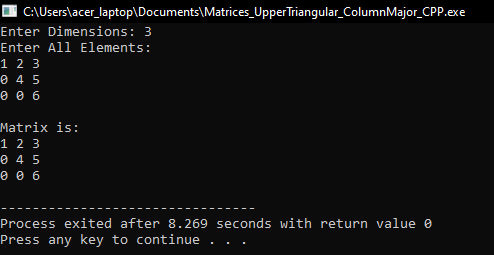In the next article, I am going to discuss Symmetric Matrix in C Language with Examples. Here, in this article, I try to explain Upper Triangular Matrix Column Major Mapping in C and C++ Language with Examples and I hope you enjoy Upper Triangular Matrix Column Major Mapping in C and C++ Language with Examples article.